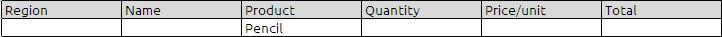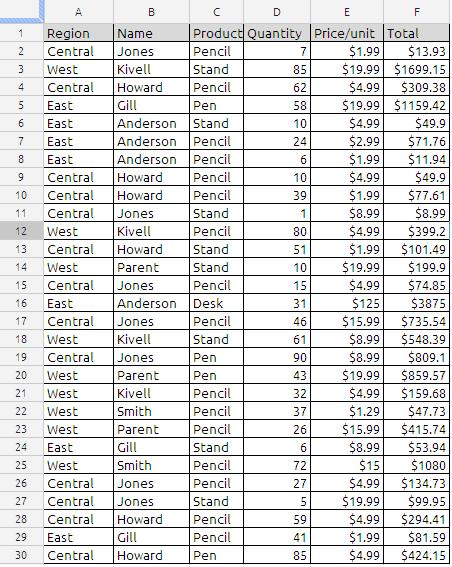# COUNTIF

Returns the number of elements that meet certain criteria within a cell range. Range is the range to which the criteria are to be applied.

COUNTIF(range, criteria)

range:  The collection containing the values to be tested. Range  is a collection that can contain any value type.

criteria:  An expression that results in a logical TRUE or FALSE. condition is an expression that can contain anything as long as the result from comparing condition to a value in range can be expressed as a Boolean value of TRUE or FALSE.

Usage Notes
Each range value is compared to criteria. If the value meets the conditional test, it is included in the count.

You can use the wildcard characters, question mark (?) and asterisk (*), in criteria. A question mark matches any single character; an asterisk matches any sequence of characters. If you want to find an actual question mark or asterisk, type a tilde (~) before the character.

Examples:
In the sample data if we want to count for the criteria “Pencil “

the formula will be
=COUNTIF(C2:C30,”Pencil”) will return value 16

the same results can be achieved using other worksheet formulas ex:
Using Sumproduct
=ArrayFormula(SUMPRODUCT((C2:C30=”Pencil”)))

Using Sum
=ArrayFormula(SUM((C2:C30=”Pencil”)))

Using DCOUNTA
=DCOUNTA(A1:F30,”Product”,K2:P3)
where K2:P3 iswith wildcard (*,?)
if we want to count the word Pen and Pencil in the countif
=COUNTIF(C2:C30,”Pen*”) will return 20
Count will include the both Pen and Pencil

To Count the unique values in the column E which contain only numbers the formula will be
=ArrayFormula(sum(1/countif(E2:E30,E2:E30))) will return with answer of 9

To Count the unique values in the column c which contain text the formula will be

=ArrayFormula(SUM((C2:C30<>””)/COUNTIF(C2:C30,C2:C30&””))) will return with value 4
this can be cross checked with =unique(c3:c30)

To count the number of cells that contain a negative number:

=COUNTIF(data,”<0″)

To count the number of cells that contain the word “yes” (not case sensitive):

=COUNTIF(range,”yes”)

To count the number of cells that contain any text:

=COUNTIF(range,”*”)

To count the number of cells that contain either “yes” or “no” (not case-sensitive):

=COUNTIF(range,”yes”)+COUNTIF(range,”no”)

To count the number of cells that contain a value between 1 and 10:

=COUNTIF(range,”>=1″)-COUNTIF(range,”>10″)

Sample data